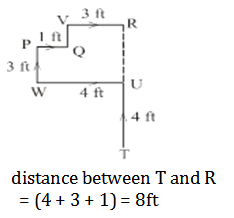Important Reasoning Questions For SSC CGL 2018-19 | Set -1

Important Reasoning Questions For SSC CGL 2018

Reasoning Questions For SSC CGL 2018. Welcome to the www.letsstudytogether.co online SSC CGL 2018 online preparation section. If you are preparing SSC CGL, CPO, RRB Railways Exams 2018, you will come across a section on “Reasoning Ability”. Here we are providing you with “Important Reasoning Questions For SSC CGL Prelims 2018”   based on the latest pattern of your daily practice.

This “Reasoning Questions For SSC CGL 2018“ is also important for other SSC & Railway exams such as CPO, CHSL, RRB Group D, ALP & Technicians Exam 2018.

Reasoning Questions For SSC CGL 2018 | Set -1

1.In each of the questions below are given two statements followed by conclusions numbered I and II. You have to take the given statements to be true even if they seen to at variance from commonalty known facts. Read all the conclusions logically follows from the given statements disregarding commonly known facts. Give answer-

Statement:
If he is intelligent
He will pass the examination
Conclusions :
I. To pass he must be intelligent
II. He will pass the examination

A. If only conclusion I follows

B.If only conclusion II follows

C.If neither conclusion I nor II follows

D.If both conclusion I and II follows

A. If only conclusion I follows

2.In each of the questions below are given two statements followed by conclusions numbered I and II. You have to take the given statements to be true even if they seen to at variance from commonalty known facts. Read all the conclusions logically follows from the given statements disregarding commonly known facts. Give answer-

Statements:
A bird in hand is worth two in the bush.
Conclusions:
I.We should be content with what we have.
II. We should not crave for what is not.

A. If only conclusion I follows

B. If only conclusion II follows

C. If neither conclusion I nor II follows

D. If both conclusion I and II follows

D.If both conclusion I and II follows

3.Find the number of triangles in the given figure.A. 16

B. 13

C. 9

D. 7

A. 16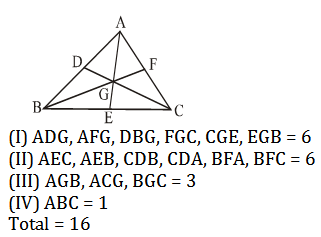4.When seen through a mirror, a clock shows 8:30. The correct time is

A. 2:30

B. 3:30

C. 5:30

D. 8:30

B. 3:30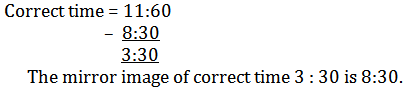5.Trace out the alternative figure which contains fig. ‘X’A. A

B. B

C. C

D. D

A. A

6.Group the given figures into three classes using each figure only once.A. 7, 8, 9; 2, 4, 3; 1, 5, 6

B. 1, 3, 2; 4, 5, 7; 6, 8, 9

C. 1, 6, 8; 3, 4, 7; 2, 5, 9

D. 1, 6, 9; 3, 4, 7; 2, 5, 8

D. 1, 6, 9; 3, 4, 7; 2, 5, 8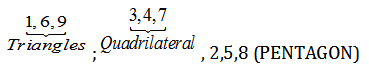7.Count the number of blocks in the given figure.A. 3

B. 4

C. 5

D. 6

C. 5

In the figure there are – 1 columns containing 2 blocks and 3 columns containing 1 block each.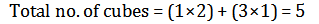8.In a dice a, b, c and d are written on the adjacent faces, in a clockwise order and e and f at the top and bottom. When C is at the top, what will be at bottom?A. A

B. B

C. C

D. Data insufficient

A. A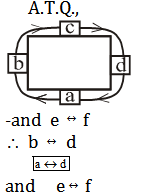9.Trace out the alternative figure which can be formed from the pieces given in fig (X)A. A

B. B

C. C

D. D

B. B

10.Maya starts at point T, walks straight to point U. Which is 4ft away. She turns left at 90° and walks to W which is 4ft away, turns 90° right and goes 3ft to P, turns 90° right and walks 1ft to Q, turns left at 90° and goes to V, which is 1ft away and once again turns 90° right and goes to R, 3ft away. What is the difference between T and R?

A. 4ft

B. 5ft

C. 7ft

D. 8ft

D.8ft